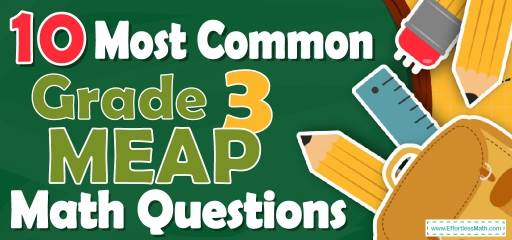# 10 Most Common 3rd Grade MEAP Math QuestionsPreparing 3rd-grade students for the MEAP Math test? Want a preview of the most common mathematics questions on the 3rd Grade MEAP Math test? If so, then you are in the right place.

The mathematics section of 3rd Grade MEAP can be a challenging area for some students, but with enough patience, it can be easy and even enjoyable!

Make sure to follow some of the related links at the bottom of this post to get a better idea of what kind of mathematics questions your students need to practice.

## 10 Sample 3rd Grade MEAP Math Practice Questions

1- Classroom A contains 8 rows of chairs with 4 chairs per row. If classroom B has three times as many chairs, which number sentence can be used to find the number of chairs in classroom B?

A. $$8 × 4 + 3$$

B. $$8 + 4 × 3$$

C. $$8 × 4 × 3$$

D. $$8 + 4 + 3$$

2- A cafeteria menu had spaghetti with meatballs for $8 and bean soup for$6. How much would it cost to buy three plates of spaghetti with meatballs and three bowls of bean soup? __________

3- There are 7 days in a week. There are 24 hours in the day. How many hours are in a week?

A. 48

B. 96

C. 168

D. 200

4- Emily described a number using these clues:
Three-digit odd numbers that have a 6 in the hundreds place and a 3 in the tens place

A. 627

B. 637

C. 632

D. 636

5- This clock shows a time after 12:00 PM. What time was it 1 hour and 30 minutes ago?

A. 12:45 PM

B. 1:45 PM

C. 1:15 PM

D. 12:15 PM

6- Olivia has 84 pastilles. She wants to put them in boxes of 4 pastilles. How many boxes does she need?

A. 20

B. 21

C. 24

D. 28

7- A football team is buying new uniforms. Each uniform costs $20. The team wants to buy 11 uniforms. Which equation represents a way to find the total cost of the uniforms? A. $$(20 × 10) + (1 × 11) = 200 + 11$$ B. $$(20 × 10) + (10 × 1) = 200 + 10$$ C. $$(20 × 10) + (20 × 1) = 200 + 20$$ D. $$(11 × 10) + (10 × 20) = 110 + 200$$ 8- There are 92 students from Lexington Elementary school at the library on Tuesday. The other 54 students in the school are practicing in the classroom. Which number sentence shows the total number of students in Riddle Elementary school? A. $$92 + 54$$ B.$$92 -54$$ C. $$92 × 54$$ D. $$92 ÷54$$ 9- Use the picture below to answer the question. Which fraction shows the shaded part of this square? A. $$\frac{92}{100}$$ B. $$\frac{92}{10}$$ C. $$\frac{90}{100}$$ D. $$\frac{8}{100}$$ 10- Which number correctly completes the number sentence $$90 × 25 =$$ ? A. 225 B. 900 C. 1250 D. 2250 ## Best 3rd Grade MEAP Math Prep Resource for 2022 ## Answers: 1- C 3times of 8 rows of chairs with 4 chairs per day is: $$3 × 8 × 4$$ 2- 42 3spaghetti with meatballs cost: $$3 × 8 = 24$$ 3bowls of bean soup cost: $$3 × 6 = 18$$ 3spaghetti with meatballs + 3bowls of bean soup cost: $$24 + 18 = 42$$ 3- C 1 day: 24 hours so 7 days $$= 7 × 24 = 168$$ hours 4- B Three-digit odd numbers that have a 6 in the hundreds place and a 3 in the tens place is 637. 632 and 636 are even numbers. 5- A Subtract hours: $$14– 1 = 13$$ Subtract the minutes: $$15 – 30 = – 15$$ The minutes are less than 0, so: • Add 60 to minutes ( $$-15 +60 =45$$ minutes) • Subtract 1 from hours $$(13 – 1 =12)$$ 6- B Olivia wants to divide 84 pastilles into boxes of 4 pastilles, so $$84 ÷ 4 = 21$$ is the number of boxes. 7- C The football team should buy 11 uniforms that each uniform cost$20 so they should pay$$(11×20) 220$$.
Therefore, choice C is correct answer:
$$(20 × 10) + (20 × 1) = 20(10+1) =20×11=220$$

8- A
Add 92 and 54 students to know the whole number of students.

9- A
the model for the fraction is divided into 100 equal parts. We shade 92 parts of these 100 parts that it’s equal to $$\frac{92}{100}$$

10- D
$$90 × 25 =2,250$$

## The Best Books to Ace the 3rd Grade MEAP Math Test

### What people say about "10 Most Common 3rd Grade MEAP Math Questions - Effortless Math: We Help Students Learn to LOVE Mathematics"?

No one replied yet.

X
24% OFF

Limited time only!

Save Over 24%

SAVE $5 It was$20.99 now it is \$15.99